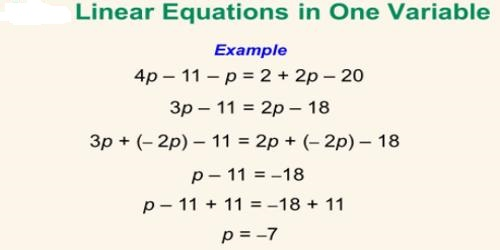Linear Equation in One Variable

Linear equation is a mathematical statement or equation that has only one variable in it. We know that to solve variables in equation number of equation should be equal to the number of the variables. In other words, if two expressions are equal to each other and you add or subtract the exact same thing to both sides, the two sides will remain equal.

Linear equation is a mathematical statement or equation that has only one variable in it. We know that to solve variables in equation number of equation should be equal to the number of the variables. So, to solve variable present in a linear equation of one variable, one equation is enough to solve the variable.Below are given some examples of linear equation in one variable:

1. 2x + 3 = 35
2. 3y + 34 = 8
3. 2z +15 = 89
4. 18x +45 = 23

Above are the examples of linear equations in one variable.

Now following are the steps used in solving a linear equation in one variable:

• Step I: Observe the linear equation carefully.
• Step II: Carefully note the quantity you need to find out.
• Step III: Divide the equation in two parts, i.e., L.H.S. and R.H.S.
• Step IV: Figure out the terms containing constants and variables.
• Step V: Transfer all the constants on the Right Hand Side (R.H.S) of the equation and variables on the Left Hand Side (L.H.S.) of the equation.
• Step VI: Perform the algebraic operations on both sides of the equation to get the value of the variable.

Let solve few examples to understand the concept in a better way.

Solve x +12 = 23.

Solution:

Let us first transfer the constants and variables on the R.H.S. and L.H.S. respectively. So,

x = 23 – 12 = x = 11.

So, value of ‘x’ is 11.

Solve 2x +13 = 43

Solution:

Transfer the constants and variables on their respective sides. So,

2x = 43 – 13 = 2x = 30

x = 30/2 = x = 15.

So, the value of ‘x’ is 15.

Note that addition and subtraction are inverse operations of each other.  For example, if you have a number that is being added that you need to move to the other side of the equation, then you would subtract it from both sides of that equation.

Information Source: